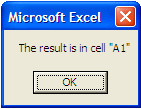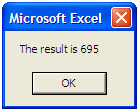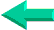# VBA Lesson 17: Message and Input Boxes (MsgBox, InputBox) in Excel

In VBA for Excel the message box (MsgBox) is the primary tool to interact with the user. For example you might want to tell the user that a long macro has finished running.

Exercise 1

Step 1: Open a new workbook and use the ALT/F11 keys to move to the Visual Basic Editor.

Step 2: Copy/Paste the following macro from here into the code window of any sheet.

Sub proLessson17a()
Sheets("Sheet1").Select
Range("A1").Value = 695
MsgBox "The macro has finished running"

End Sub

Notice the space following MsgBox and the use of quotation marks surrounding the text

Step 3: Use the ALT/F11 keys to go back to Excel and run the macro proLessson17a.

The value 695 is entered in cell A1 and the following message box appears.Step 4: Delete the macro in the Visual Basic Editor and the value 695 from cell A1

Exercise 2

You might want to tell the user where he will find the result.

Step 1: Use the ALT/F11 keys to move to the Visual Basic Editor.

Step 2: Copy/Paste the following macro from here into the code window of any sheet.

Sub proLessson17b()
Sheets("Sheet1").Select
Range("A1").Value = 695

MsgBox "The result is in cell ""A1"""

End Sub

Notice the space following MsgBox, the use of quotation marks surrounding the text and the double quotation mars around A1 because we want the address to show on the message box between quotation marks.

Step 3: Use the ALT/F11 keys to go back to Excel and run the macro proLessson17b.

The value 695 is entered in cell A1 and the following message box appearsStep 4: Delete the macro in the Visual Basic Editor and the value 695 from cell A1

Exercise 3

Instead of telling the user that the value is in cell A1, you might want to tell him what the result is in the message box itself.

Step 1: Use the ALT/F11 keys to move to the Visual Basic Editor.

Step 2: Copy/Paste the following macro from here into the code window of any sheet.

Sub proLessson17c()
Sheets("Sheet1").Select
Range("A1").Value = 695

MsgBox "The result is " & Range("A1").Value

End Sub

Notice the space following MsgBox, the use of quotation marks surrounding the text, the space at the end of the text and the spaces surrounding the ampersand.

Step 3: Use the ALT/F11 keys to go back to Excel and run the macro proLessson17c.

The value 695 is entered in cell A1 and the following message box appearsStep 4: Close Excel without saving anything.

You can use the message box to inform the user. You might also ask the user (with a Yes/No message box) if he is sure that he wants a certain critical procedure to run (deleting things).

There are many types of message boxes (information, alert, exclamation or questions. Then if you need an input from the user you will start using the input box.

Go to the next lesson
Lesson
18 : VBA to work with  Excel DatabasesBack home